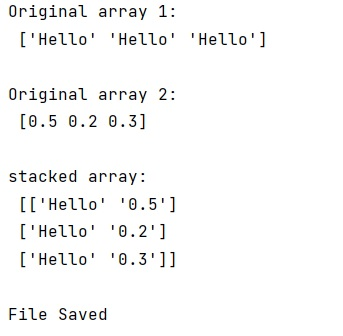# How to use numpy.savetxt() to write strings and float number to an ASCII file?

Learn, how to use numpy.savetxt() to write strings and float number to an ASCII file?
Submitted by Pranit Sharma, on January 16, 2023

NumPy is an abbreviated form of Numerical Python. It is used for different types of scientific operations in python. Numpy is a vast library in python which is used for almost every kind of scientific or mathematical operation. It is itself an array which is a collection of various methods and functions for processing the arrays.

## Using numpy.savetxt() to write strings and float number to an ASCII file

Suppose that we are given two NumPy arrays containing float and string values respectively. We need to stack these two arrays together and write them into a text file of columns.

For this purpose, we will use numpy.savetxt() method. First, we will create two arrays containing string and float values respectively and then we will stack them together. Finally, we will pass this stacked array into the numpy.savetxt() method as an argument.

Let us understand with the help of an example,

## Python code to use numpy.savetxt() to write strings and float number to an ASCII file

```# Import numpy
import numpy as np

# Import pandas
import pandas as pd

# Creating two numpy arrays
arr1 = np.array(['Hello', 'Hello', 'Hello'])
arr2 = np.array([ 0.5,0.2,0.3])

# Display original arrays
print("Original array 1:\n",arr1,"\n")
print("Original array 2:\n",arr2,"\n")

# Creating a stacked array
arr = np.column_stack((arr1,arr2))

# Display stacked array
print("stacked array:\n",arr,"\n")

# Saving the array into file
np.savetxt('test.txt', arr, delimiter=" ", fmt="%s")

# Print a message
print("File Saved")
```

Output:What's New (MCQs)

Top Interview Coding Problems/Challenges!

IncludeHelp's Blogs

Languages: » C » C++ » C++ STL » Java » Data Structure » C#.Net » Android » Kotlin » SQL
Web Technologies: » PHP » Python » JavaScript » CSS » Ajax » Node.js » Web programming/HTML
Solved programs: » C » C++ » DS » Java » C#
Aptitude que. & ans.: » C » C++ » Java » DBMS
Interview que. & ans.: » C » Embedded C » Java » SEO » HR
CS Subjects: » CS Basics » O.S. » Networks » DBMS » Embedded Systems » Cloud Computing
» Machine learning » CS Organizations » Linux » DOS
More: » Articles » Puzzles » News/Updates